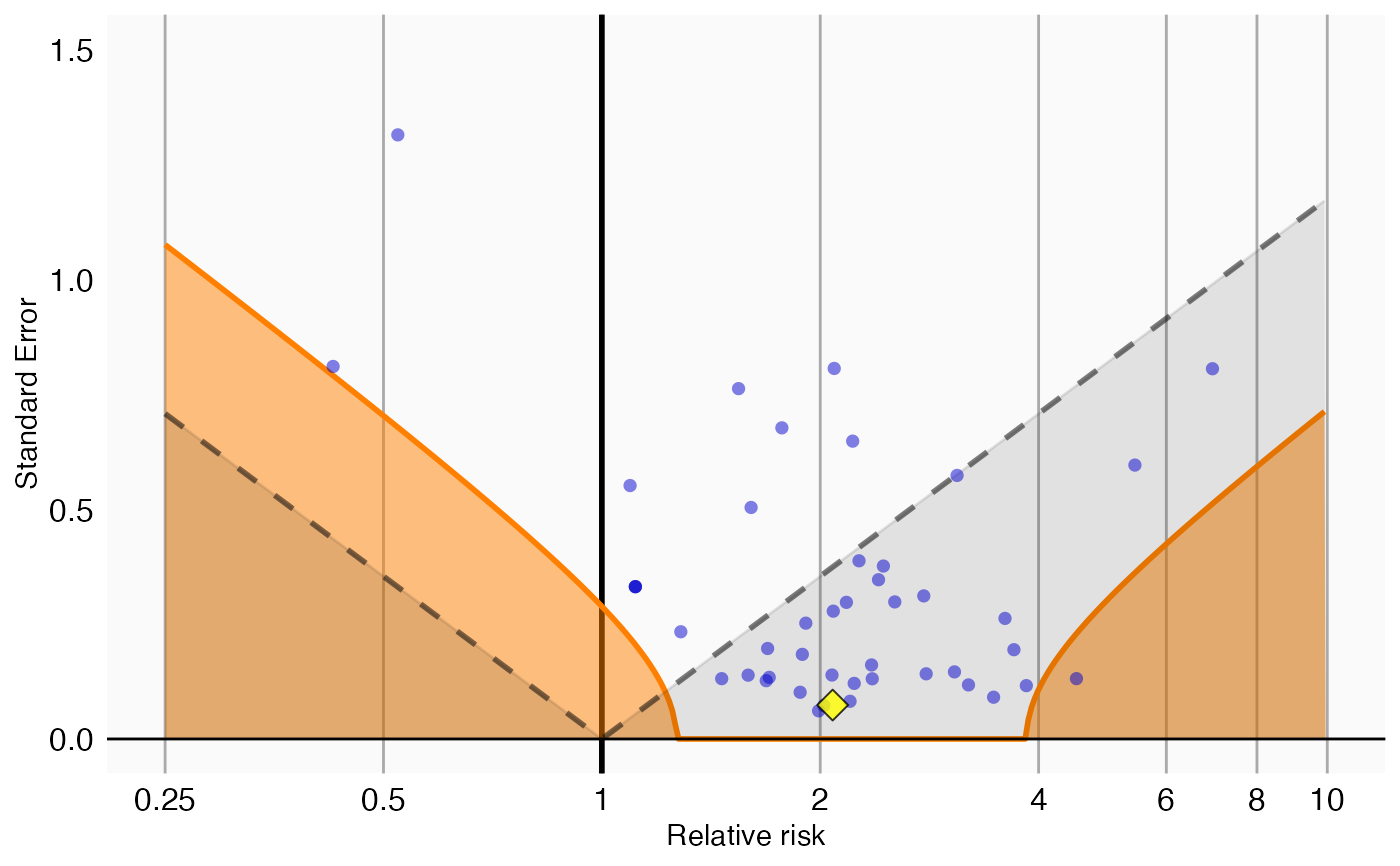plotCalibrationEffect creates a plot showing the effect of the calibration.

plotCalibrationEffect(
logRrNegatives,
seLogRrNegatives,
logRrPositives = NULL,
seLogRrPositives = NULL,
null = NULL,
alpha = 0.05,
xLabel = "Relative risk",
title,
showCis = FALSE,
showExpectedAbsoluteSystematicError = FALSE,
fileName = NULL,
xLimits = c(0.25, 10),
yLimits = c(0, 1.5)
)

## Arguments

logRrNegatives

A numeric vector of effect estimates of the negative controls on the log scale.

seLogRrNegatives

The standard error of the log of the effect estimates of the negative controls.

logRrPositives

Optional: A numeric vector of effect estimates of the positive controls on the log scale.

seLogRrPositives

Optional: The standard error of the log of the effect estimates of the positive controls.

null

An object representing the fitted null distribution as created by the fitNull or fitMcmcNull functions. If not provided, a null will be fitted before plotting.

alpha

The alpha for the hypothesis test.

xLabel

The label on the x-axis: the name of the effect estimate.

title

Optional: the main title for the plot

showCis

Show 95 percent credible intervals for the calibrated p = alpha boundary.

showExpectedAbsoluteSystematicError

Show the expected absolute systematic error. If null is of type mcmcNull the 95 percent credible interval will also be shown.

fileName

Name of the file where the plot should be saved, for example 'plot.png'. See the function ggsave in the ggplot2 package for supported file formats.

xLimits

Vector of length 2 for limits of the plot x axis - defaults to 0.25, 10

yLimits

Vector of length 2 for size limits of the y axis - defaults to 0, 1.5

## Value

A Ggplot object. Use the ggsave function to save to file.

## Details

Creates a plot with the effect estimate on the x-axis and the standard error on the y-axis. Negative controls are shown as blue dots, positive controls as yellow diamonds. The area below the dashed line indicated estimates with p < 0.05. The orange area indicates estimates with calibrated p < 0.05.

## Examples

data(sccs)
negatives <- sccs[sccs$groundTruth == 0, ] positive <- sccs[sccs$groundTruth == 1, ]
plotCalibrationEffect(negatives$logRr, negatives$seLogRr, positive$logRr, positive$seLogRr)
#> Warning: Removed 1 rows containing missing values (geom_vline).